# Bags

The first bag has a weight of 18 kg, the second bag is a one-third lighter than the first and the third bag has three times less the weight of all three bags together. Determine their weights.

a =  18 kg
b =  12 kg
c =  15 kg

### Step-by-step explanation:

c = (18+12+c)/3

2c = 30

c = 15

Our simple equation calculator calculates it.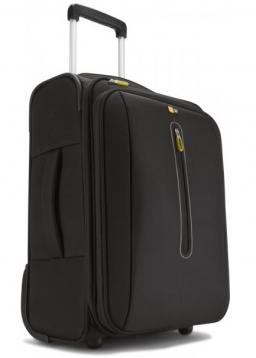Did you find an error or inaccuracy? Feel free to write us. Thank you!Tips to related online calculators
Do you have a linear equation or system of equations and looking for its solution? Or do you have a quadratic equation?

## Related math problems and questions:

• Potatoes bags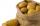I have three bags with 21 kg potatoes. The first bag is 5.5 kg more than in the second bag and the third is 0.5 kg more than in the second bag. Determine how many kgs of potatoes is in each bag.
• Barrel 3Barrel with water weights 118 kg. When we get off 75% of water, it weights 35 kg. How many kg has an empty barrel?
• WeightsMarry and John together weighing 49 kg. Their weights are in ratio 1:6. Determine their weights.
• Copper partsFrom copper cast were made 3 parts. At first consumed one third of casting, the second 2/3 of rest and the third weighed 18 kg. What is the weight of original copper cast?
• Two melons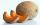There are 2 melons in the box. Together they weigh 11kg. The weight of green is 3 kg less than three times the weight of yellow. What is the weight of a green melon?
• HomeworkMother and Kate together weigh 24.5 kg more than the father. Father weighs 7.6 kg more than mother. Father weight 44.8 kg more than Kate. Determine weights of father mother and Kate.
• Bag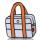Nelly found interesting point. An empty bag weighs 4 kg less than full. And empty bag is 5 times easier than full. How many kg weigh things in this bag?
• Empty canisterThe canister is filled with oil weight 17 kg. If filled only half weight 9 kg. What weight does my empty canister have?
• Reservoir + waterThe reservoir filled with water weighs 12 kg and after pouring off three-quarters of the water weights 3 kg. Calculate the weight and volume of the reservoir.
• Water wessel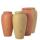The vessel containing water has a weight 19 kg. After pouring half the water its weigth 11 kg. What is the weight of the empty vessel?
• Vegan shopTo the shops brought together 23.2 kg of goods, fruits, vegetables and nuts. fruit was 4.7 kg more than vegetables, nuts was 1.5 kg less than the fruit. Determine the amount of fruits, vegetables and nuts.
• Balance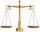The rod are 1.9 m long hanging weights 4 kg and 1 kg on ends. Where are centre of rod (distance from weight 4 kg) to be in balance?
• Container with water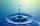The weight of a container with water is 2.48 kg. If the cast 75% water, the container with water has a weight of 0.98 kg. Determine the weight of the empty container. How much water was originally in the container?
• PotatoesFor three days the store sold 1400 kg of potatoes. The first day they sold 100 kilograms of potatoes less than the second day, the third-day three-fifths of what they sold the first day. How many kgs of potatoes sold every day?
• MixingIf we mix 5 kg of goods of one kind and 3 kg second one, resulting mixture cost 16.50 EUR/kg. If these quantities are mixed in reverse - first three kilograms and 5 kilograms second cost of mixture is 18.50 EUR/kg. What is the price of one kg of goods of
• Lorry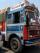The lorry was loaded with 18 boxes of 15 kg. How many boxes with weight 18 kg can be loaded, if total load must be same?
• Three numbersFind three numbers so that the second number is 4 times greater than the first and the third is lower by 5 than the second number. Their sum is 67.